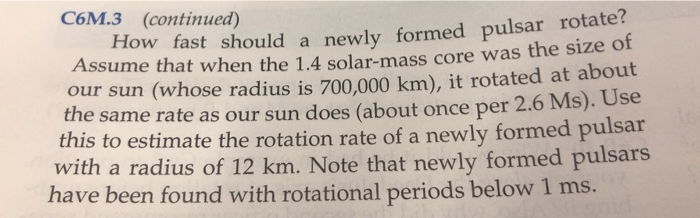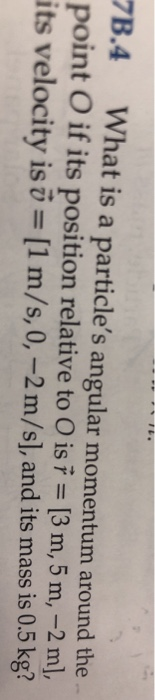# Question Solved1 AnswerC6M.3 (continued) How fast should a newly formed pulsar rotate? Assume that when the 1.4 solar-mass core was the size of our sun (whose radius is 700.000 km), it rotated at about the same rate as our sun does (about once per 2.6 Ms). Use this to estimate the rotation rate of a newly formed pulsar with a radius of 12 km. Note that newly formed pulsars have C6M.3 (continued) How fast should a newly formed pulsar rotate? Assume that when the 1.4 solar-mass core was the size of our sun (whose radius is 700.000 km), it rotated at about the same rate as our sun does (about once per 2.6 Ms). Use this to estimate the rotation rate of a newly formed pulsar with a radius of 12 km. Note that newly formed pulsars have been found with rotational periods below 1 ms. 78.4 What is a particle's angular momentum around the point O if its position relative to O is = [3 m, 5 m, –2 m], its velocity is o = [1 m/s, 0, -2 m/s], and its mass is 0.5 kg?Transcribed Image Text: C6M.3 (continued) How fast should a newly formed pulsar rotate? Assume that when the 1.4 solar-mass core was the size of our sun (whose radius is 700.000 km), it rotated at about the same rate as our sun does (about once per 2.6 Ms). Use this to estimate the rotation rate of a newly formed pulsar with a radius of 12 km. Note that newly formed pulsars have been found with rotational periods below 1 ms. 78.4 What is a particle's angular momentum around the point O if its position relative to O is = [3 m, 5 m, –2 m], its velocity is o = [1 m/s, 0, -2 m/s], and its mass is 0.5 kg?
More
Transcribed Image Text: C6M.3 (continued) How fast should a newly formed pulsar rotate? Assume that when the 1.4 solar-mass core was the size of our sun (whose radius is 700.000 km), it rotated at about the same rate as our sun does (about once per 2.6 Ms). Use this to estimate the rotation rate of a newly formed pulsar with a radius of 12 km. Note that newly formed pulsars have been found with rotational periods below 1 ms. 78.4 What is a particle's angular momentum around the point O if its position relative to O is = [3 m, 5 m, –2 m], its velocity is o = [1 m/s, 0, -2 m/s], and its mass is 0.5 kg?